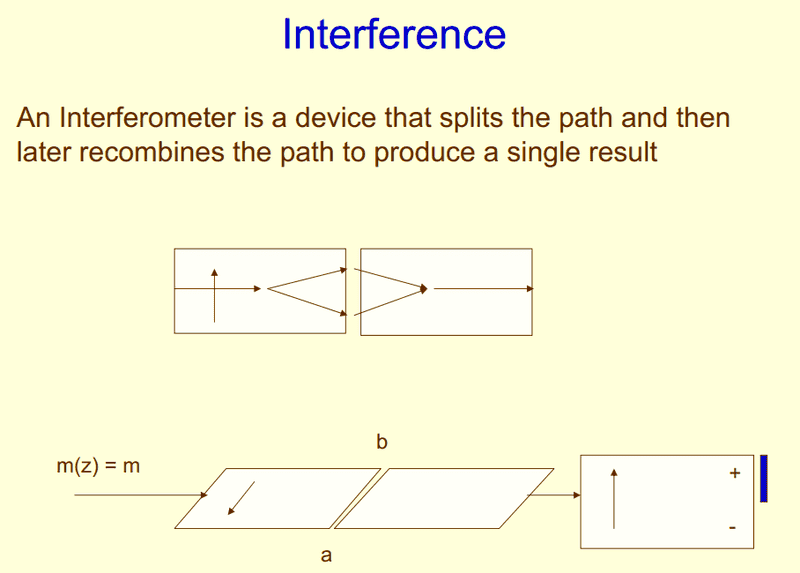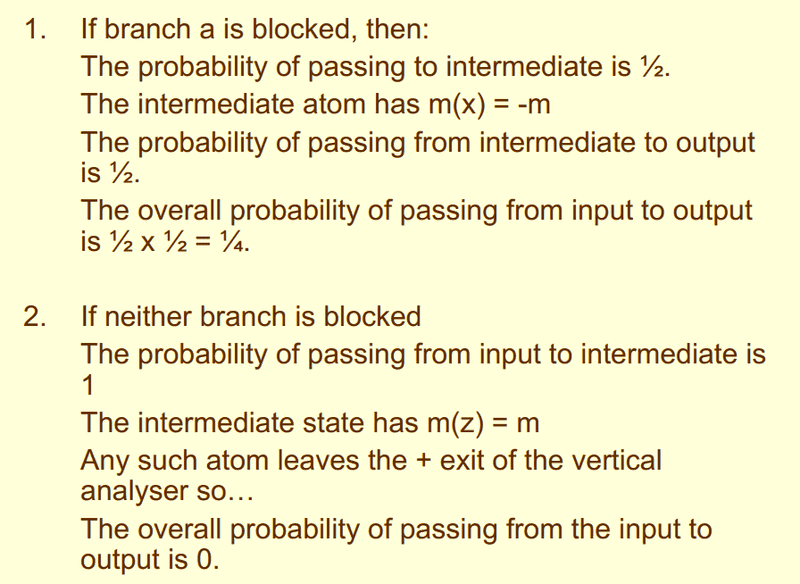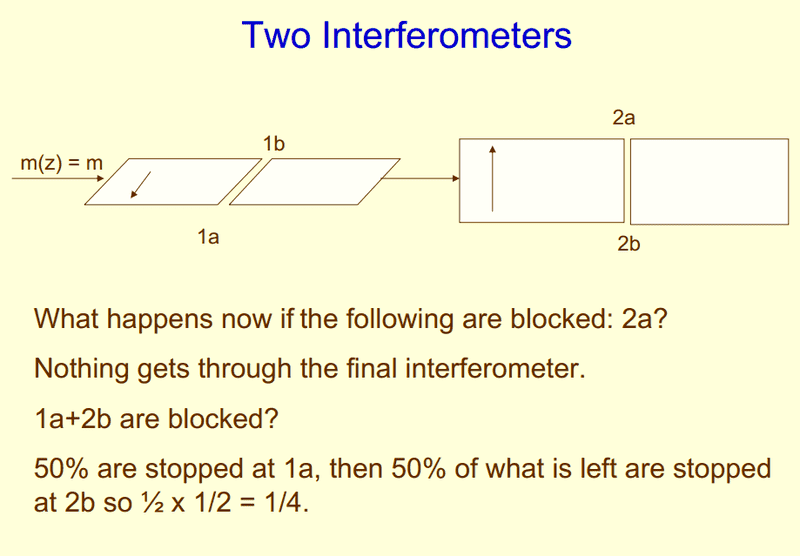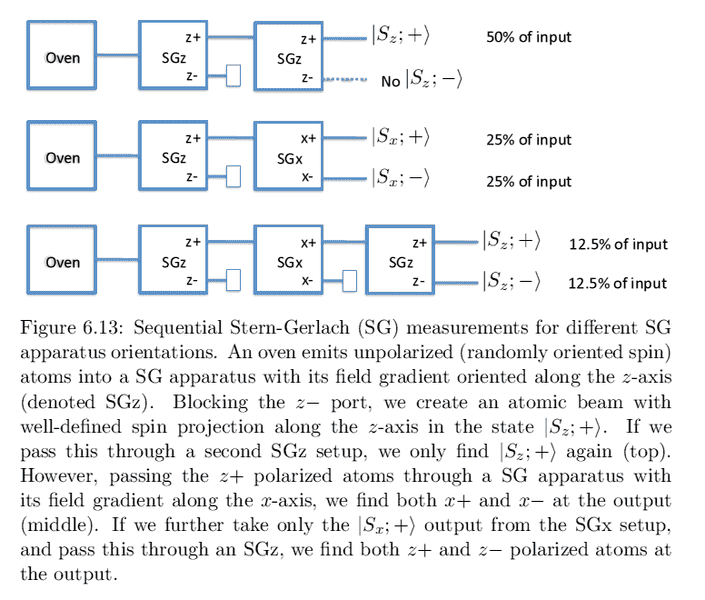# Stern-Gerlach Inferometer (I don't understand the lecturer)

## Homework Statement

This isn't exactly a homework question, but rather from the lecturer's slides. I'm not sure if I understand what's going on.## The Attempt at a Solution

Branch a is blocked

1. Atoms all with +ve spin enter
2. all the atoms can approach the terminal a (+)
3. Just before crossing over the gap, half tries to go through a(+), half tries to go through b(-). factor = 1/2
4. atoms passes through b, +ve spin gets converted into -ve spin
5. second analyser at 90o, so splits into cos2(90/2) = 1/2 with +ve spin, splits into 1 - cos2(90/2) = sin2(90/2) 1/2 with -ve spin factor = 1/2
6. Result: 1/4 with -ve spin, 1/4 with positive spin. Ones with +ve spin approaches and crashes at blue barrier. Ones with -ve spin approaches below. Result = 1/4 -ve spin.

Neither Branch is blocked

1. atoms approach a(+)
2. Just before crossing over, splits into a(+) and (b-); factor = 1/2
3. Recombines. factor becomes 1.
4. second analyser at 90o, so splits into cos2(90/2) = 1/2 with +ve spin, splits into 1 - cos2(90/2) = sin2(90/2) 1/2 with -ve spin factor = 1/2
5. Result: 1/2 with -ve spin, 1/2 with positive spin. Ones with +ve spin approaches and crashes at blue barrier. Ones with -ve spin approaches below. Result = 1/2 -ve spin.

But, from the slides I can infer that going to an inferometer that's 90 degrees does not change the particle's spin at all..if it's all +ve heading towards a perpendicular inferometer, it remains +ve.

However, this seems to contradict what i'm currently reading, which is also notes provided by the same lecturer. It suggests that passing from a z-plane to a x-plane stern-gerlach apparatus appears to 'reset' the spins back to having both +ve and -ve.mfb
Mentor
But, from the slides I can infer that going to an inferometer that's 90 degrees does not change the particle's spin at all..if it's all +ve heading towards a perpendicular inferometer, it remains +ve.
90° relative to what?
The spin is not changed if the initial spin is aligned with the polarization axis of the interferometer.
The spin is changed if there is an angle between both.

90° relative to what?
The spin is not changed if the initial spin is aligned with the polarization axis of the interferometer.
The spin is changed if there is an angle between both.

What I meant was suppose the atom (initially with +spin) travels from a z-plane GS to an x-plane GS (Which is 90o to the z-plane), the atom now has 2 spins, both +ve and -ve but with a factor of cos2(α/2) in the +ve spin, sin2(α/2), in the -ve spin where α is the angle between plane.

mfb
Mentor
(initially with +spin)
In which direction?
travels from a z-plane GS to an x-plane GS (Which is 90o to the z-plane), the atom now has 2 spins, both +ve and -ve but with a factor of cos2(α/2) in the +ve spin, sin2(α/2), in the -ve spin where α is the angle between plane.
Right.

In which direction?

In the z+ direction.

1. If branch a is blocked, why does the intermediate atom have -m spin? Is it because it passes through b? Does this mean that each time it crosses a gap it acquires a spin that is either +ve/-ve, depending on where it passes through?

mfb
Mentor
1. If branch a is blocked, why does the intermediate atom have -m spin? Is it because it passes through b?
Right.
Does this mean that each time it crosses a gap it acquires a spin that is either +ve/-ve, depending on where it passes through?
Right

Ok, I think I have resolved it!

1. First of all, i wrongly assumed that the atom having +m(z) is the same as +m(x). I didn't realize that they are different axes. If no branches are blocked, the interferometer does nothing to the atom, regardless of it's orientation.

2. Initial stage, we have no clue whether the atom is +m(x) or -m(x) oriented. The only way to tell is to see where it passes through - (If it passes through a, it must be +m(x) then, if it passes through b, it must be -m(x) then.) We have no information beforehand. So factor = 1/2.

3. Suppose a is blocked. Then anything passes through b will be of -m(x). Knowing precisely its spin is -m(x), we can't determine its spin in m(z) (Weird I know). When it passes into the final GS, it can either be +m(z) or -m(z) (again, we can only tell after it passes through the output). So factor is 1/2.

4. Now if neither branch is blocked, we know clearly that the atom is +m(z) since the interferometer does nothing to the atom. But we have no information on its m(x) spin. Now certain of it being +m(z), it must pass through the + terminal at the final GS. Hence 0 probability.

5. Now consider this scenario, where we "explicitly" evaluate probabilities at a point. The atom can either go through a or b. Then it can either go through + or - at the final GS. P(through a) = 1/4, P(through b) = 1/4. So total probability = 1/2. But, the problem here lies with the assumption that the atom "either goes through a or b", because since we know for definite that its spin is +m(z), we cannot say what its spin in m(x) is (Not unless you observe it throughout)

6. It appears that when you observe the atom entirely (passing through a/b, entering the GS). The probability of the atom passing from the input to the output is 1/2. But when you if you just let the atom do whatever it wants without watching (interacting) it, the probability is 0. So clearly, an atom behaves differently when it's being watched than it being unwatched.

Last edited: